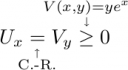# Add Explanation to LaTeX Formulas (Equations)

When writing a document that contains mathematics, many time the need to add an explanation (e.g. stating the theorem used) is raised. To answer this need I wrote two short LaTeX macros: \explain and \explainup.

\newcommand{\explain}{\underset{\mathclap{\overset{\uparrow}{#2}}}{#1}}
\newcommand{\explainup}{\overset{\mathclap{\underset{\downarrow}{#2}}}{#1}}


This macros add the explanation below and above the formula.
The first argument is the relation (actually it can be anything) that needs be explained, for example =. The second argument is the actual explanation.

The macros depend on the mathtools package by

#### Example

\documentclass{article}
\usepackage{mathtools}
\makeatletter

\newcommand{\explain}{\underset{\mathclap{\overset{\uparrow}{#2}}}{#1}}
\newcommand{\explainup}{\overset{\mathclap{\underset{\downarrow}{#2}}}{#1}}

\makeatother
\begin{document}

$U_x\explain{=}{\textrm{C.-R.}}V_y\explainup{\geq}{V(x,y)=ye^x}0$

\end{document}


Will result in## 4 thoughts on “Add Explanation to LaTeX Formulas (Equations)”

1.Kiran SIngh

Nice one, txs for this.

2.Ronen

That’s grate, Thanks!

But, do you know how I do the following:
\left(\explain{\frac{a}{b}}{\mbox{{\rm The brace wont expand!}}}+c\right)

Here, the () Don’t take the width of the “math” part (without the text in the “explain”) but.. the hight of the explain block (the arrow and the text). Except the ugliness, it make the text unreadable – He intersect with the ( ).
Do you know how I prevent the () to raise?

Thanks,
Ronen.

3.Guy Post author

Hi Ronen,

A quick solution would be to use manual brace size

\bigg(\explain{\frac{a}{b}}{\mbox{{\rm The brace wont expand!}}}+c\bigg)

I currently don’t have a better solution, as the explain environment must be have its full size (or else multiple lines will be clamped together), but the width of the description must be declared as zero (so no extra space will be introduced).

4.Ronen

Thanks.

This site uses Akismet to reduce spam. Learn how your comment data is processed.